# ISEE Upper Level Math : How to find the length of an edge of a cube

## Example Questions

### Example Question #1 : How To Find The Length Of An Edge Of A Cube

The volume of a cube is. What is the length of an edge of the cube?Explanation:

Letbe the length of an edge of the cube. The volume of a cube can be determined by the equation: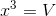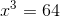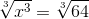### Example Question #2 : How To Find The Length Of An Edge Of A Cube

There is a sculpture in front of town hall which is shaped like a cube. If it has a volume of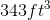, what is the length of one side of the cube?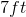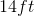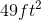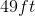Explanation:

There is a sculpture in front of town hall which is shaped like a cube. If it has a volume of, what is the length of one side of the cube?

To find the side length of a cube from its volume, simply use the following formula: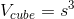Plug in what is known and use some algebra to get our answer: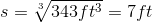### Example Question #3 : How To Find The Length Of An Edge Of A Cube

You have a cube with a volume of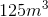. What is the cube's side length?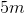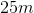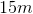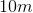Explanation:

You have a cube with a volume of. What is the cube's side length?

If we begin with the formula for volume of a cube, we can work backwards to find the side length.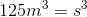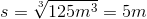### Example Question #4 : How To Find The Length Of An Edge Of A Cube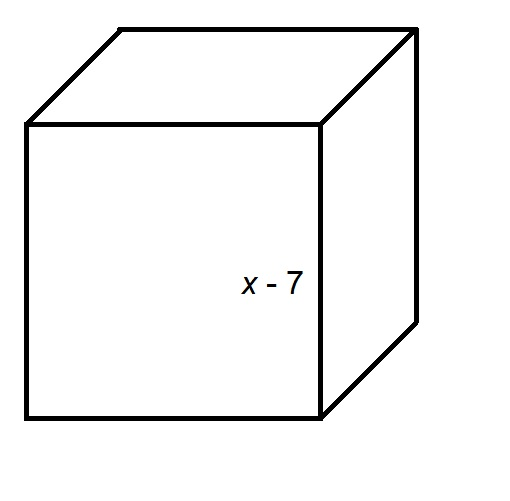The above cube has surface area 486. Evaluate.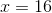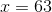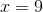Explanation:

The surface area of a cube is six times the square of the length of each edge, which here is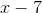. Therefore,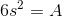Substituting, then solving for: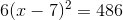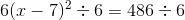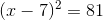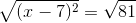Since the sidelength is positive,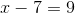### Example Question #5 : How To Find The Length Of An Edge Of A Cube

You have a crate with equal dimensions (height, length and width). If the volume of the cube is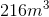, what is the length of one of the crate's dimension?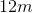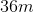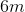Not enough information to solve the equation.Explanation:

You have a crate with equal dimensions (height, length and width). If the volume of the cube is, what is the length of one of the crate's dimension?

Let's begin with realizing that we are dealing with a cube. A crate with equal dimensions will have equal height, length, and width, so it must be a cube.

With that in mind, we can find our side length by starting with the volume and working backward.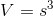So, to find our side length, we just need to take the cubed root of the volume.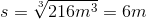So, our answer is 6 meters, a fairly large crate!

### Example Question #6 : How To Find The Length Of An Edge Of A Cube

If the volume of a cube is 30, what must be the length of the edge of the cube?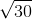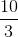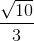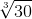Explanation:

Write the formula to find the volume of a cube.Substitute the volume into the equation.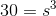Cube root both sides.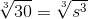The answer is:### Example Question #7 : How To Find The Length Of An Edge Of A Cube

You have a cube with a volume of. What is the cube's side length?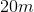Explanation:

You have a cube with a volume of. What is the cube's side length?

If we begin with the formula for volume of a cube, we can work backwards to find the side length.